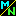Navigation Panel:(These buttons explained below)Question Corner and Discussion Area

# The Sum of the Geometric Series 1 + 1/2 + 1/4 + · · ·

Asked by Krishna Srinivasan on Friday Dec 22, 1995:
My name is Krishna. I'm now in Grade 12. When I was in Grade 11, I saw a question in the math club.

Actually, I have already asked a similar question like the one below, but this one is little different.

The question is,

What is the value of, 1 + (1/2) + (1/4) + (1/8) ...... ?

How can you find a definite value for an answer when it keeps on continuing?

Thank you.

Krishna

The reason an infinite sum like 1 + 1/2 + 1/4 + · · · can have a definite value is that one is really looking at the sequence of numbers
1
1 + 1/2 = 3/2
1 + 1/2 + 1/4 = 7/4
1 + 1/2 + 1/4 + 1/8 = 15/8
etc.,

and this sequence of numbers (1, 3/2, 7/4, 15/8, . . . ) is converging to a limit. It is this limit which we call the "value" of the infinite sum.

How do we find this value?

If we assume it exists and just want to find what it is, let's call it S. Now

S = 1 + 1/2 + 1/4 + 1/8 + · · ·
so, if we multiply it by 1/2, we get
(1/2) S = 1/2 + 1/4 + 1/8 + 1/16 + · · ·
Now, if we subtract the second equation from the first, the 1/2, 1/4, 1/8, etc. all cancel, and we get S - (1/2)S = 1 which means S/2 = 1 and so S = 2.

This same technique can be used to find the sum of any "geometric series", that it, a series where each term is some number r times the previous term. If the first term is a, then the series is

S = a + a r + a r^2 + a r^3 + · · ·
so, multiplying both sides by r,
r S = a r + a r^2 + a r^3 + a r^4 + · · ·
and, subtracting the second equation from the first, you get S - r S = a which you can solve to get S = a/(1-r). Your example was the case a = 1, r = 1/2.

In using this technique, we have assumed that the infinite sum exists, then found the value. But we can also use it to tell whether the sum exists or not: if you look at the finite sum

S = a + a r + a r^2 + a r^3 + · · · + a r^n
then multiply by r to get
rS = a r + a r^2 + a r^3 + a r^4 + · · · + a r^(n+1)
and subtract the second from the first, the terms a r, a r^2, . . . , a r^n all cancel and you are left with S - r S = a - a r^(n+1), soAs long as |r| < 1, the term r^(n+1) will go to zero as n goes to infinity, so the finite sum S will approach a / (1-r) as n goes to infinity. Thus the value of the infinite sum is a / (1-r), and this also proves that the infinite sum exists, as long as |r| < 1.

In your example, the finite sums were

1 = 2 - 1/1
3/2 = 2 - 1/2
7/4 = 2 - 1/4
15/8 = 2 - 1/8
and so on; the nth finite sum is 2 - 1/2^n. This converges to 2 as n goes to infinity, so 2 is the value of the infinite sum.

[ Submit Your Own Question ] [ Create a Discussion Topic ]

This part of the site maintained by (No Current Maintainers)
Last updated: April 19, 1999
Original Web Site Creator / Mathematical Content Developer: Philip Spencer
Current Network Coordinator and Contact Person: Joel Chan - mathnet@math.toronto.edu

Navigation Panel: